KNOWLEDGEBASE - ARTICLE #2172

# Prism 8.4 does not allow certain multiple comparisons choices in two-way repeated measures ANOVA when sphericity is not assumed

With the release of Prism 8.4, Prism no longer lets you choose certain multiple comparisons tests after two-way ANOVA when the data are repeated measures and you chose not to assume sphericity but rather to use the Geisser-Greenhouse correction. In these situations, the multiple comparisons results reported by Prism 8.0 to 8.3 were incorrect. Prism 8.0 introduced the option when performing a repeated measures two-way ANOVA to assume sphericity of the analyzed data, or to use the method of Geisser and Greenhouse to correct for violations of this assumption. However, for some combinations of options, Prism was not taking the repeated measures into account when calculating certain multiple comparisons tests. As a result, these specific tests used an incorrect error term and/or had an incorrect number of degrees of freedom (df).

The ANOVA results are fine. The issue is only with multiple comparisons following repeated measures two-way ANOVA when you don't assume sphericity. In these cases the multiple comparisons really don't make sense unless you make an alternative assumption. As explained at the bottom of this page, we are planning a future version of Prism that will offer choices of assumptions (covariation matrices). For now, some multiple comparisons options are disabled.

## Which multiple comparisons tests were disabled?

The four situations were Prism 8.4 disables multiple comparisons are summarized in the table below and detailed in the following screenshots.

Matching by which factor(s)? Which multiple comparisons were disabled?
Each row represents a different time point, so matched values are stacked into a subcolumn
• Compare column means (main column effect)
Each column represents a different time point, so matched values are spread across a row
• Compare row means (main row effect)
Matched values are spread across a row AND matched values are stacked into a subcolumn
• Compare column means (main column effect)
• Compare row means (main row effect)

### Case 1:

Matching by which factor(s)?: Each row represents a different time point, so matched values are stacked into a subcolumn.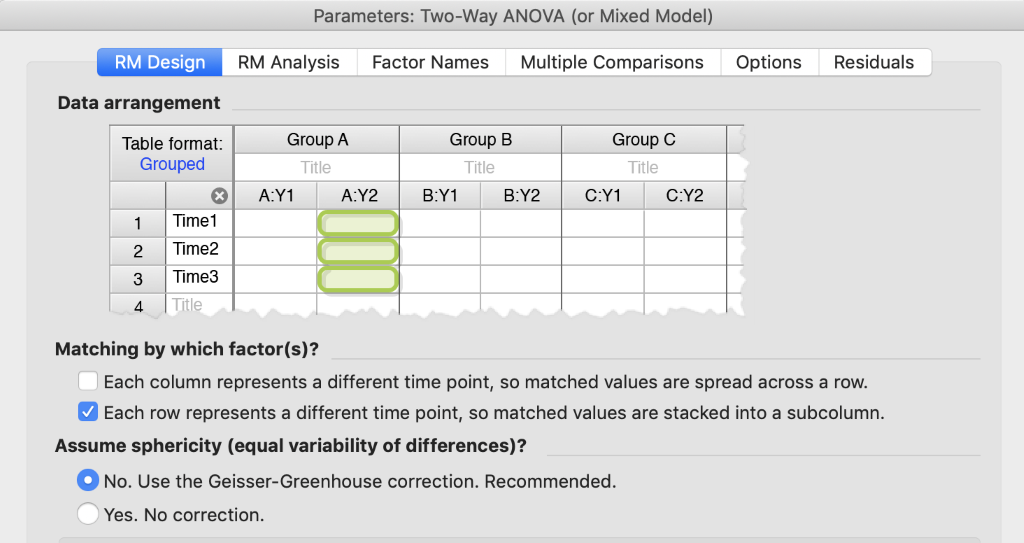Disabled multiple comparisons test: Compare column means (main column effect)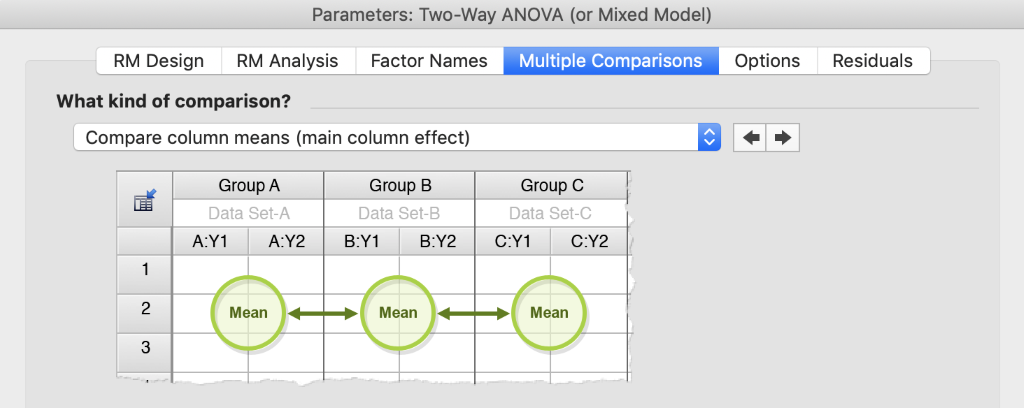### Case 2:

Matching by which factor(s)?: Each column represents a different time point, so matched values are spread across a row.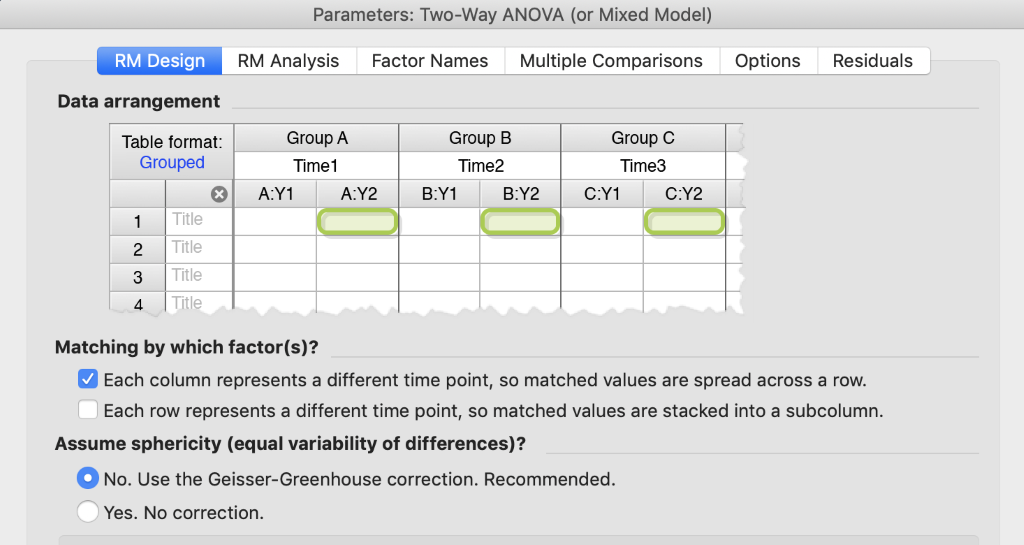Disabled multiple comparisons test: Compare row means (main row effect)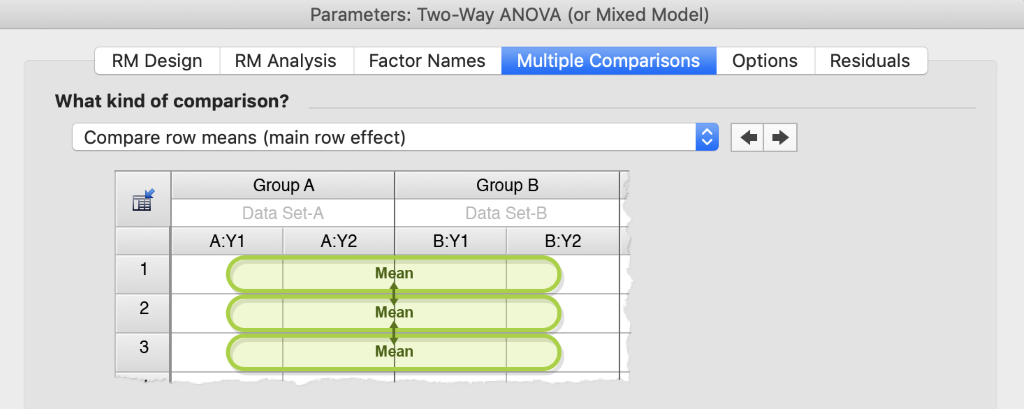### Case 3:

Matching by which factor(s)?: Each column represents a different time point, so matched values are spread across a row. AND Each row represents a different time point, so matched values are stacked into a subcolumn.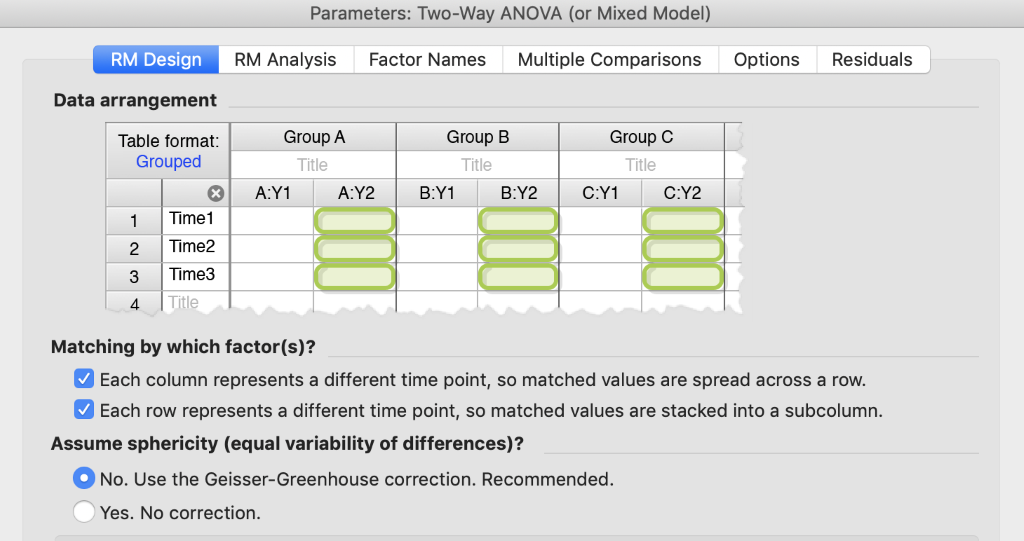Disabled multiple comparisons test: Compare column means (main column effect)### Case 4:

Matching by which factor(s)?: Each column represents a different time point, so matched values are spread across a row. AND Each row represents a different time point, so matched values are stacked into a subcolumn.Disabled multiple comparisons test: Compare row means (main row effect)## Fixed bug

While addressing the situations described above, a bug was discovered and fixed in 8.4.

Matching by which factor(s)?: Each column represents a different time point, so matched values are spread across a row.Multiple comparisons test with updated results: Within each column, compare rows (simple effects within columns)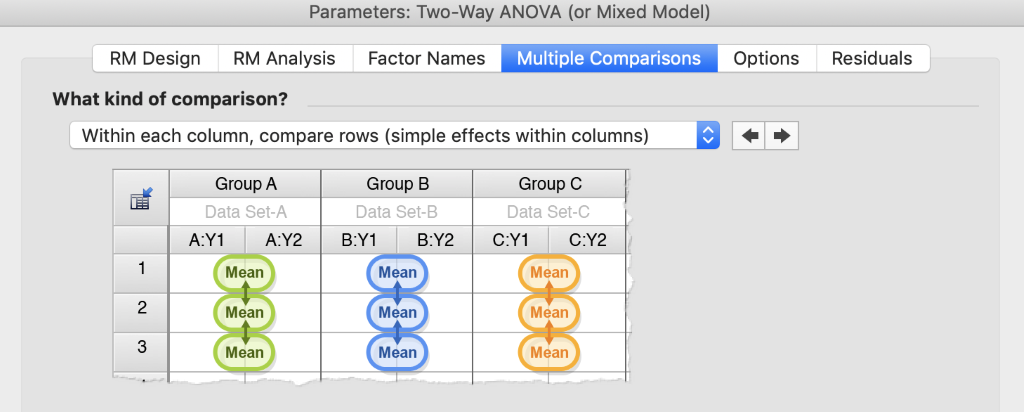## What Prism Assumes for the Covariance Structure in Repeated Measures Designs

ANOVA estimates both the effect sizes and the error or noise in the experiment so the effect sizes can be compared to something (think signal to noise ratio). The residual error is simply the difference between the observed value and the model prediction. In the simplest form of ANOVA, this error is assumed to be the same throughout the experiment (regardless of the treatment combination) and these errors are assumed to be independent of each other. In an experiment where repeated measures are made on the same subject, the observations can no longer be assumed to be independent, and as a result, we usually see correlations in the residual errors. Instead of assuming a constant error variance with no correlations, we need to specify or assume a covariance structure for the residuals in a repeated measures experiment.

The simplest covariance structure that accounts for correlated errors is compound symmetry. Here, the correlation of the errors between time points within a subject are assumed to be the same regardless of how distant in time the repeated measures are. With this structure, the variance at each time point is also assumed to be the same. This is the structure Prism 8 assumes when "Yes" is selected under "Assume sphericity". When you choose "No" under "Assume sphericity", the covariance structure allows for unique correlations for each pair of time points and does not assume equal variation at each time point. In this scenario, the multiple comparison tests are challenging because you can't use data from the entire experiment to estimate the error for a single comparison. Sometimes, a comparison can't be made, and when it can, the power can be very poor. Other covariance structures, such as the autoregressive lag 1, assume correlations are highest for adjacent time points and systematically decrease as the distance between time points increases. This structure also assumes equal variation at each time point, but note, it is only appropriate for evenly spaced time intervals. Prism 8 does not offer this covariance structure, but we are considering offering it, and possibly others, in a future release. This would allow us to offer reasonable multiple comparison tests after repeated measures two-way ANOVA.

## Explore the Knowledgebase

Analyze, graph and present your scientific work easily with GraphPad Prism. No coding required.